Question

(1 point) In this problem you will calculate the area between f(x) = x2 and the...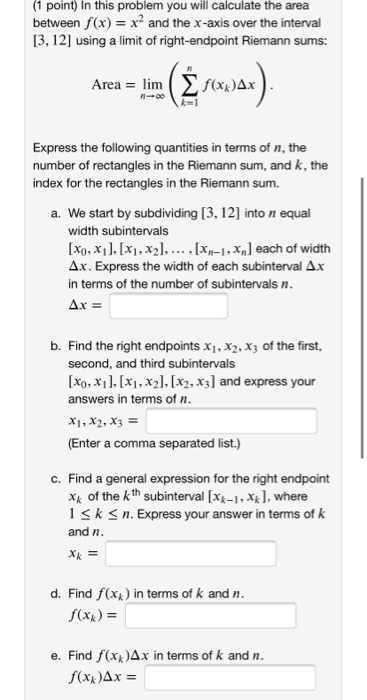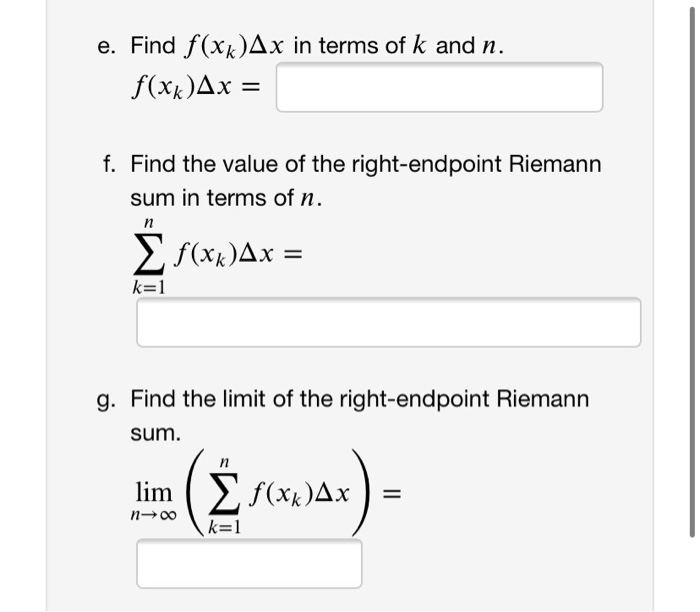(1 point) In this problem you will calculate the area between f(x) = x2 and the x-axis over the interval [3,12] using a limit of right-endpoint Riemann sums: Area = lim ( f(xxAx bir (3 forwar). Express the following quantities in terms of n, the number of rectangles in the Riemann sum, and k, the index for the rectangles in the Riemann sum. a. We start by subdividing [3, 12) into n equal width subintervals [x0, x1], [x1, x2),..., [Xn-1, x, each of width Ax. Express the width of each subinterval Ax in terms of the number of subintervals n. Ax = b. Find the right endpoints X1, X2, X3 of the first, second, and third subintervals [x0, x1], [x1, x2], [X2, X3] and express your answers in terms of n. X1, X2, X3 = (Enter a comma separated list.) c. Find a general expression for the right endpoint Xk of the kth subinterval [Xk-1, xx], where 1 sk <n. Express your answer in terms of k and n. Xx = d. Find f(x) in terms of k and n. f(x) = e. Find f (xx)Ax in terms of k and n. f(x)Ax =
e. Find f(xk)Ax in terms of k and n. f(xk)Ax = f. Find the value of the right-endpoint Riemann sum in terms of n. n f(xx)Ax = k=1 g. Find the limit of the right-endpoint Riemann sum. lim ( f(xx)Ax = n00 k=1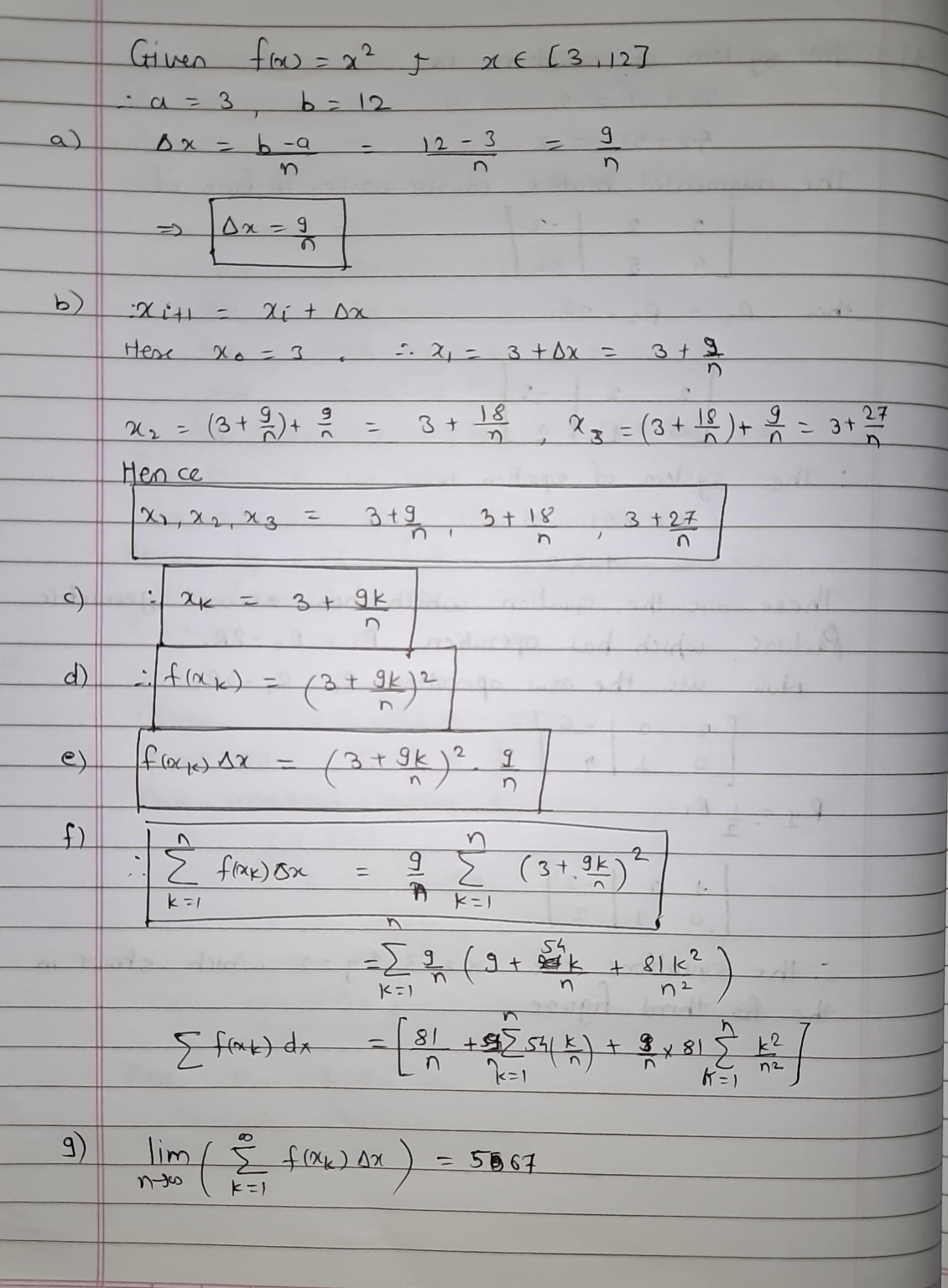Earn Coins

Coins can be redeemed for fabulous gifts.

Similar Homework Help Questions
• Estimate the area of the region bounded by the graph of f(x)-x + 2 and the x-axis on [0,4] in the following ways a. Divide [0,4] into n = 4 subintervals and approximate the area of the region using...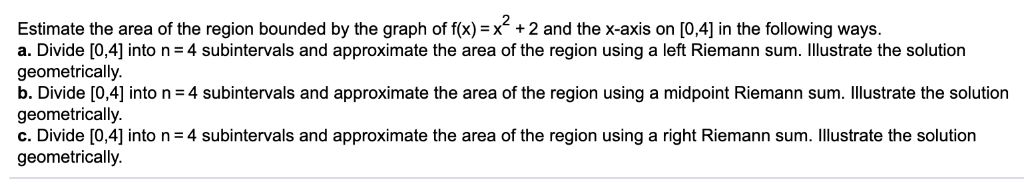Estimate the area of the region bounded by the graph of f(x)-x + 2 and the x-axis on [0,4] in the following ways a. Divide [0,4] into n = 4 subintervals and approximate the area of the region using a left Riemann sum. Illustrate the solution geometrically. b. Divide [0,4] into n = 4 subintervals and approximate the area of the region using a midpoint Riemann sum· illustrate the solution geometrically. C. Divide  into n = 4 subintervals and...

• Please answer with work Graph the function f(x) over the given interval. Partition the interval into...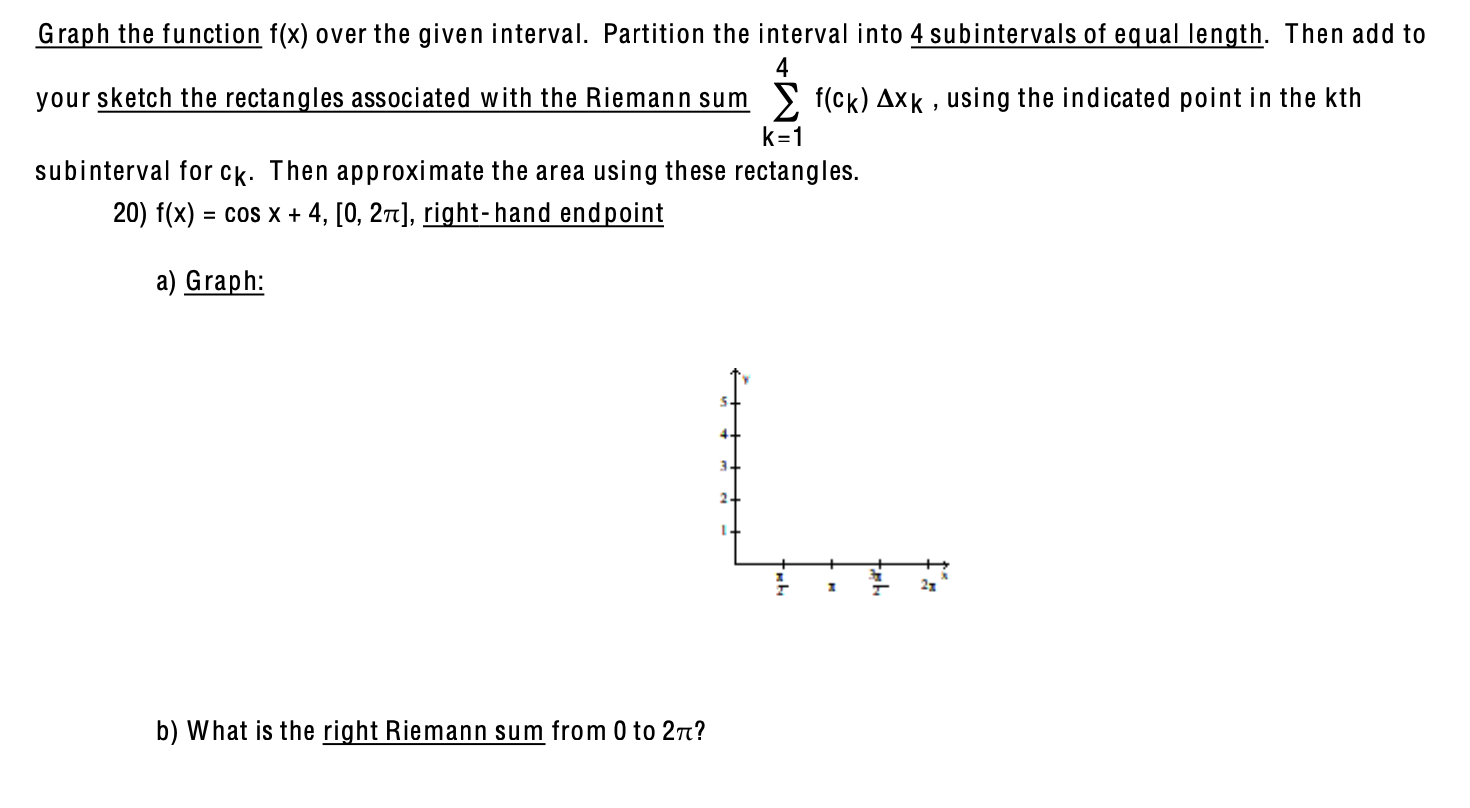Please answer with work Graph the function f(x) over the given interval. Partition the interval into 4 subintervals of equal length. Then add to 4 your sketch the rectangles associated with the Riemann sum f(ck) Axk, using the indicated point in the kth k=1 subinterval for ck. Then approximate the area using these rectangles. 20) f(x) = cos x + 4, [0, 2TT), right-hand endpoint a) Graph: 2 7 22 b) What is the right Riemann sum from 0 to...

• Let f(x) = x on the interval [1,2]. Let the interval be divided into two equal...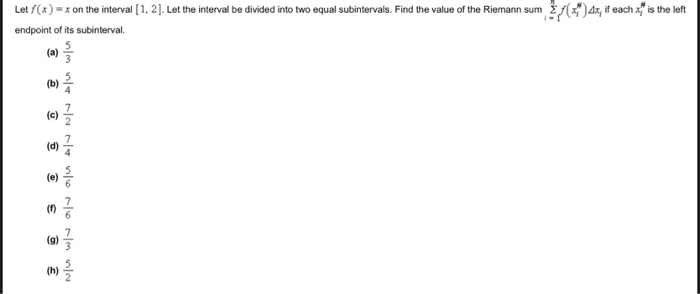Let f(x) = x on the interval [1,2]. Let the interval be divided into two equal subintervals. Find the value of the Riemann sum endpoint of its subinterval / dx, if each X;" is the left

• 3. Find the sum of the areas of approximating rectangles for the area under f(x) =...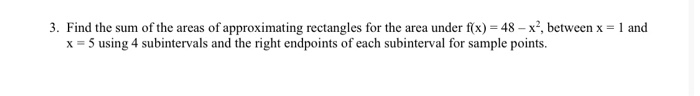3. Find the sum of the areas of approximating rectangles for the area under f(x) = 48 - x?, between x = 1 and x = 5 using 4 subintervals and the right endpoints of each subinterval for sample points.

• 2. Write the limit of the Riemann sums as a definite integral. plz !!! Cancel 1....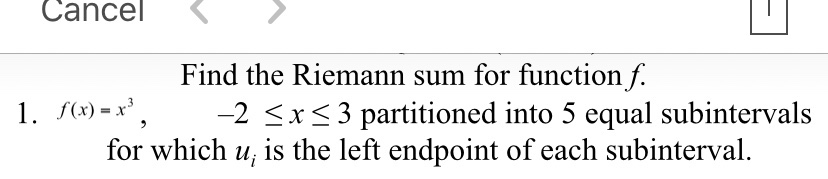2. Write the limit of the Riemann sums as a definite integral. plz !!! Cancel 1. f(x) = x3 Find the Riemann sum for function f. -2 < x < 3 partitioned into 5 equal subintervals for which u; is the left endpoint of each subinterval. 9 1 • dx a. 성 - 1 b. Sutra ( + r + 6)dx - 3 2. C. { (-6x (-6x3 - 3x² + 2x)dx -2

• 3. Find the sum of the areas of approximating rectangles for the area under f(x) =...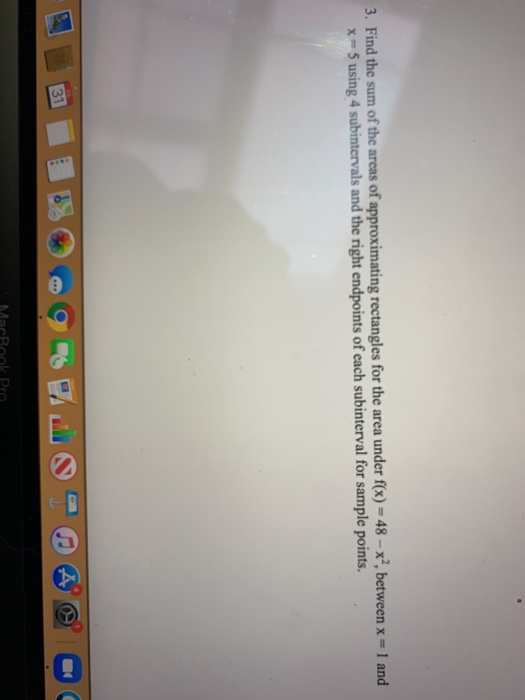3. Find the sum of the areas of approximating rectangles for the area under f(x) = 48 - x?, between x = 1 and x = 5 using 4 subintervals and the right endpoints of each subinterval for sample points. 31

• 4 Graph the function f(x) = cos x on the interval ( - 1,1], showing the...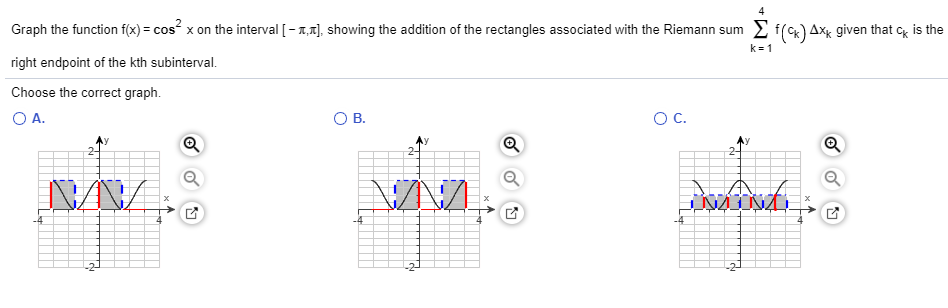4 Graph the function f(x) = cos x on the interval ( - 1,1], showing the addition of the rectangles associated with the Riemann sum Ef() 4x4 given that ck is the right endpoint of the kth subinterval. Choose the correct graph. O C. OA OB. 1 NA VN/ 2

• Use finite approximation to estimate the area under the graph of f(x) =x2 and above the...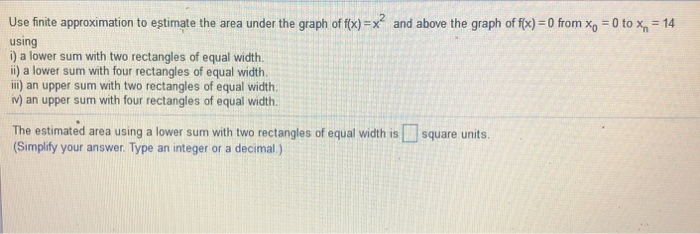Use finite approximation to estimate the area under the graph of f(x) =x2 and above the graph of f(x) = 0 from x0-0 to xn-14 using i) a lower sum with two rectangles of equal width. ii) a lower sum with four rectangles of equal width ili) an upper sum with two rectangles of equal width iv) an upper sum with four rectangles of equal width. The estimated area using a lower sum with two rectangles of equal width is...

• 5. (12 pts.) Consider the region bounded by f(x) 4-2x and the x-axis on interval [-1,...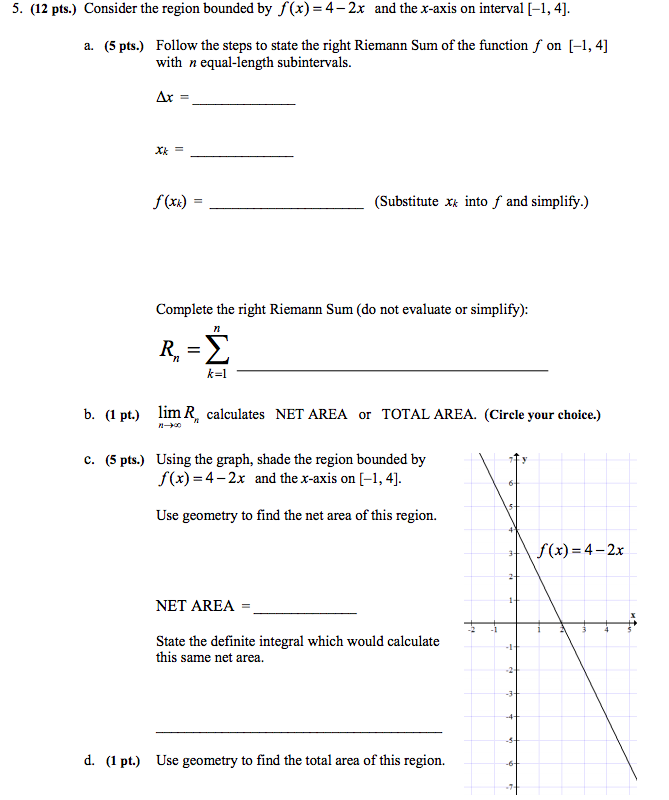5. (12 pts.) Consider the region bounded by f(x) 4-2x and the x-axis on interval [-1, 4] Follow the steps to state the right Riemann Sum of the function f with n equal-length subintervals on [-, 4] (5 pts.) a. Xk= f(xa) (Substitute x into f and simplify.) Complete the right Riemann Sum (do not evaluate or simplify): -2 b. (1 pt.) lim R calculates NET AREA or TOTAL AREA. (Circle your choice.) Using the graph, shade the region bounded...

• (6) Evaluate the Riemann sum for f(x) = x2 + 2x – 1, 1 < x...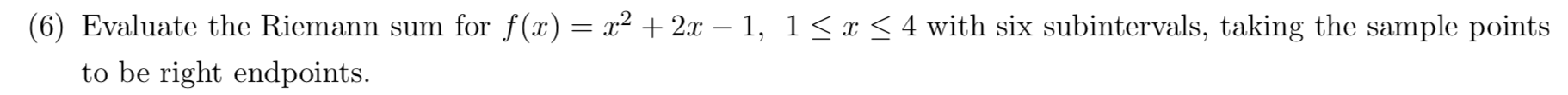(6) Evaluate the Riemann sum for f(x) = x2 + 2x – 1, 1 < x < 4 with six subintervals, taking the sample points to be right endpoints.